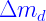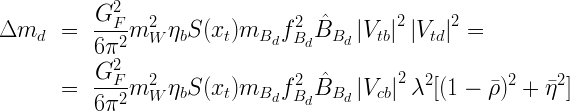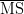## Constraint fromIn the Standard Model,oscillations occur through a second order process (a box diagram) with a loop containing W and up-type quarks. The box diagram with the top quark gives the dominant contribution. Theoscillation frequency, which is related to the mass difference between the light and the heavy mass eigenstates of the system is expressed, in the Standard Model, as function ofand the other elements of CKM matrix:whereis the Inami-Lim function and,is thetop mass,is the W mass, andis the perturbative QCD short distance NLO correction. The remaining factorencodes the information of non-perturbative QCD. The experimental values we use are summarized in the Table of Inputs. The representation of this constraint in theplane is given below.EPS - PDF - PNG - JPG - GIF

Ideas, requests, problems regarding this web site? Send feedback